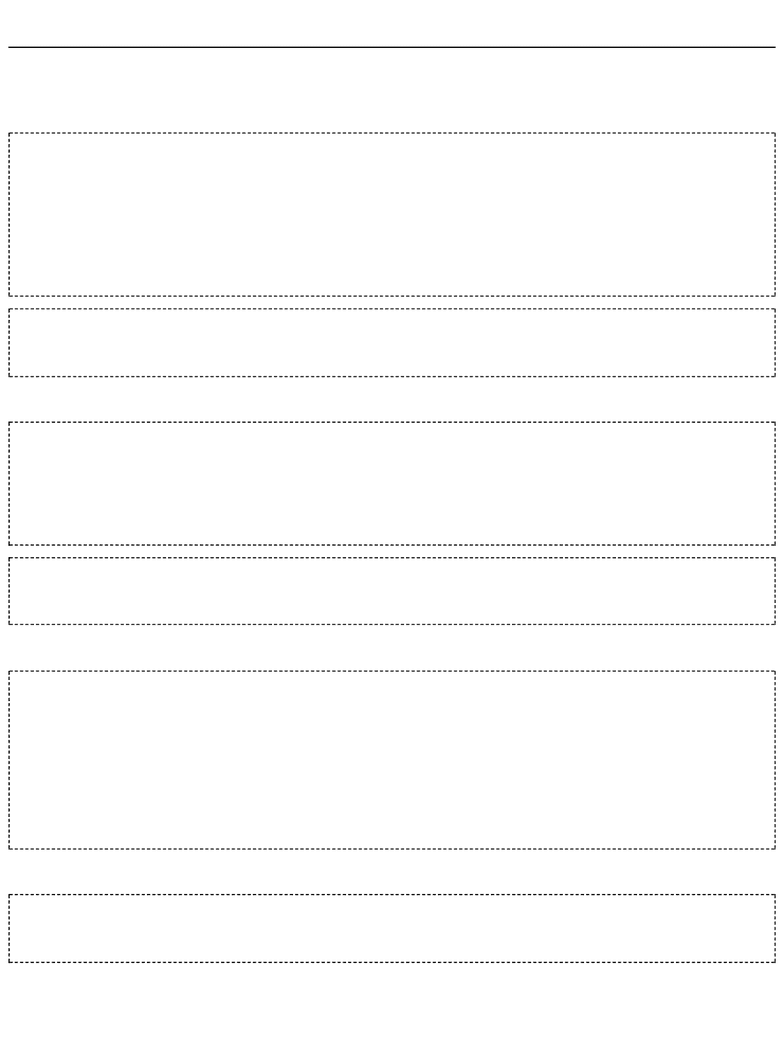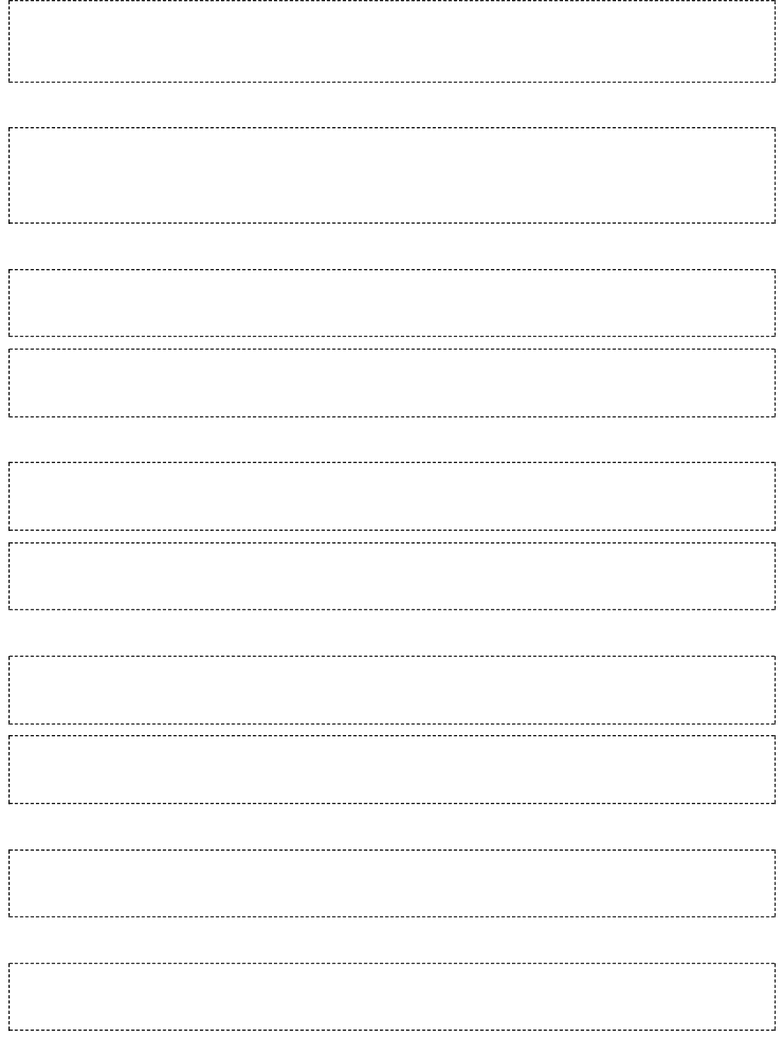Study Guides (390,000)
US (220,000)
Purdue (3,000)
CS (100)
All (10)
Midterm

# CS 17700 Study Guide - Midterm Guide: Infinite Loop, Binary Number, Graded RingExam

Department
Computer Sciences
Course Code
CS 17700
Professor
All
Study Guide
Midterm

This preview shows pages 1-2. to view the full 6 pages of the document.Midterm 1
Note: Correct answer is marked with a *
Q1. Which numbers are printed by the following loop:
sum = 0
x = 10
while x > 1 :
sum = sum + x
x = x - 3
print sum
(A) 22
(B) *21
(C) 0
(D) 20
Q2. Consider the following code snippet. What is the output of calling: fun(10, 20, “BOB”)?
def fun(a, b, c):
if (a < b or c == "A"):
if(a < 0 and c == "B"):
return a + b
else:
print a - b
else:
return -1
(A) *-10
(B) 30
(C) -1
(D) 0
Q3. Consider of the following code snipet:
def change(picture):
width = getWidth(picture)
height = getHeight(picture)
mid = height/2
for x in range(0,width):
for y in range (0,height):
if ( mid < y ):
setColor(getPixel(picture,x,y),blue)
else:
setColor(getPixel(picture,x,y),red)
return picture
Which of the following is CORRECT. (Assume the width and height of the picture are even numbers)
(A) The upper half of the picture will be blue
(B) The picture will be all red
(C) The picture will be all blue
(D) *The upper half of the picture will be red
Q4. Which of the following functions takes and changes the pixels having pure red color to black? (i)

Only pages 1-2 are available for preview. Some parts have been intentionally blurred.def removeRed1(picture):
myColor = makeColor(255, 0, 0)
for p in getPixels(picture):
setColor(p, myColor)
(ii)
def removeRed2(picture):
for x in range(0,getWidth(picture)):
for y in range (0, getHeight(picture)):
p =getPixelAt(picture, x,y)
if getRed(p) < 100:
setRed(getPixel(picture, x, y),0)
(iii)
def removeRed3(picture):
for p in getPixels(picture):
if getColor(p) == red :
setColor(p, black)
(A) i
(B) ii
(C) *iii
(D) i,ii
Q5.What is the result of the following function?
def myFun(picture):
for p in getPixels(picture):
value= (getRed(p)+getGreen(p)+getBlue(p))/3
setColor(p,makeColor(value, value, value))
(A) Creates a negative from picture
(B) Makes the colors in picture darker
(C) Makes the colors in picture lighter
(D) *Converts picture to greyscale
Q6. Which is the output of the following function?
def func():
myList = [11, 34, 71, 93, 11, 27, 11]
myList.remove(11);
print myList
(A) [34, 71, 93, 27]
(B) [11, 34, 71, 93, 11, 27]
(C) * [34, 71, 93, 11, 27, 11]
(D) [11, 34, 71, 93, 27]
Q7. Consider the following function:
def func2():
string1 = "halley's comet"
string2 = "this is a picture of a house"
print string2[:a].capitalize() + string1[:b].capitalize()
What should be the values of a and b such that the output of the function is “This is Halley”?
(A) * 8, 6
(B) 7, 5
(C) 9, 5
(D) 6, 6